﻿ 基于牛顿法及拟牛顿法的非线性规划算法改进及实证研究

# 基于牛顿法及拟牛顿法的非线性规划算法改进及实证研究The Improvement and Empirical Study of Nonlinear Programming Algorithm Based on Newton Method and Quasi-Newton Method

Abstract: Nonlinear programming has always been a hot topic in the research of optimization theory. In this paper, three commonly used methods for solving unconstrained nonlinear programming (gradient method, Newton method and quasi-Newton method) are compared and analyzed. Two new algorithms are given by improving the original method, and the convergence of the improved algorithm is illustrated. On this basis, this paper makes an empirical study on the location of distribution center based on competition.

1. 引言

$\mathrm{min}f\left( X \right)$

2. 梯度法

$\nabla f{\left({X}^{\left(k+1\right)}\right)}^{\text{T}}\cdot \nabla f\left({X}^{\left(k\right)}\right)=0$Figure 1. The iteration roadmap of Gradient method

$\mathrm{min}f\left(X\right)=-2{x}_{1}{x}_{2}-2{x}_{2}+{x}_{1}^{2}+2{x}_{2}^{2}$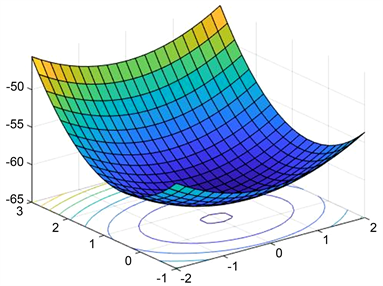Figure 2. Function image of the objective function in example 1

${X}^{\left(0\right)}={\left(0,0\right)}^{\text{T}}$ 出发，沿方向 ${d}^{\left(0\right)}=-\nabla f\left({X}^{\left(0\right)}\right)=\left(\begin{array}{c}0\\ 2\end{array}\right)$ 进行一维搜索， ${t}_{0}=\frac{1}{4}$，在直线上的极大点

${X}^{\left(1\right)}={X}^{\left(0\right)}+{t}_{0}{d}^{\left(0\right)}=\left(\begin{array}{c}0\\ 0\end{array}\right)+\frac{1}{4}\left(\begin{array}{c}0\\ 2\end{array}\right)=\left(\begin{array}{c}0\\ \frac{1}{2}\end{array}\right)$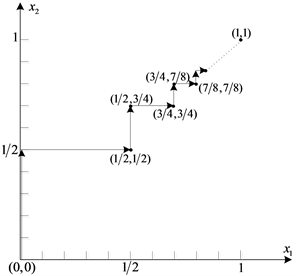Figure 3. The iteration point and iteration roadmap of example 1 were solved by gradient methodTable 1. Initial value points and iteration table of example 1 were solved by gradient method

3. 牛顿法

$f\left(X\right)\approx g\left(X\right)=f\left({X}^{\left(k\right)}\right)+\nabla f{\left({X}^{\left(k\right)}\right)}^{\text{T}}\left(X-{X}^{\left(k\right)}\right)+\frac{1}{2}{\left(X-{X}^{\left(k\right)}\right)}^{\text{T}}{\nabla }^{2}f\left({X}^{\left(k\right)}\right)\left(X-{X}^{\left( k \right)}\right)$

$\nabla f\left({X}^{\left(k\right)}\right)+{\nabla }^{2}f\left({X}^{\left(k\right)}\right)\left(X-{X}^{\left(k\right)}\right)=0$

${X}^{\left(k+1\right)}={X}^{\left(k\right)}-{\left[{\nabla }^{2}f\left({X}^{\left(k\right)}\right)\right]}^{-1}\nabla f\left({X}^{\left( k \right)}\right)$

${d}^{\left(k\right)}=-{\left[{\nabla }^{2}f\left({X}^{\left(k\right)}\right)\right]}^{-1}\nabla f\left({X}^{\left( k \right)}\right)$

${X}^{\left(k+1\right)}={X}^{\left(k\right)}+{d}^{\left( k \right)}$

$\mathrm{min}f\left(X\right)=-2{x}_{1}{x}_{2}-2{x}_{2}+{x}_{1}^{2}+2{x}_{2}^{2}$

Hessian阵 $H\left(X\right)={\nabla }^{2}f\left(X\right)=\left(\begin{array}{cc}2& -2\\ -2& 4\end{array}\right)$ 为正定矩阵(即目标函数为二次凸函数)。

$H\left({X}^{\left(0\right)}\right)={\nabla }^{2}f\left({X}^{\left(0\right)}\right)=\left(\begin{array}{cc}2& -2\\ -2& 4\end{array}\right)$$H{\left({X}^{\left(0\right)}\right)}^{-1}=\left(\begin{array}{cc}1& \frac{1}{2}\\ \frac{1}{2}& \frac{1}{2}\end{array}\right)$

${X}^{\left(1\right)}={X}^{\left(0\right)}-H{\left({X}^{\left(0\right)}\right)}^{-1}\nabla f\left({X}^{\left(0\right)}\right)=\left(\begin{array}{c}0\\ 0\end{array}\right)-\left(\begin{array}{cc}1& \frac{1}{2}\\ \frac{1}{2}& \frac{1}{2}\end{array}\right)\left(\begin{array}{c}0\\ -2\end{array}\right)=\left( 1 1 \right)$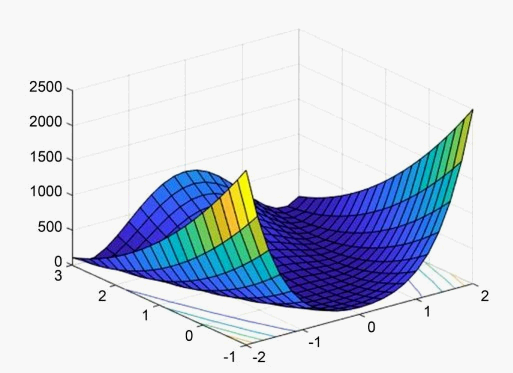Figure 4. Function image of the test functionTable 2. Initial value points and iteration table of the test function were solved by the three methods

GN算法的算法步骤：

(1) 给出初始点 ${X}^{\left(0\right)}\in {E}^{n}$，精度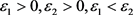，令 $k=0$

(2) 计算 $\nabla f\left({X}^{\left(k\right)}\right)$

(3) 若 $‖\nabla f\left({X}^{\left(k\right)}\right)‖<{\epsilon }_{1}$，则迭代结束，取 $\stackrel{¯}{X}={X}^{\left(k\right)}$，否则转(4)；

(4) 若 $‖\nabla f\left({X}^{\left(k\right)}\right)‖\ge {\epsilon }_{1}$

(I)若 $‖\nabla f\left({X}^{\left(k\right)}\right)‖\ge {\epsilon }_{2}$，用一维搜索求 $\phi \left(t\right)=f\left({X}^{\left(k\right)}+t{d}^{\left(k\right)}\right)$ 的一个极小点 ${t}_{k}$，使 $f\left({X}^{\left(k\right)}+{t}_{k}{d}^{\left(k\right)}\right)，其中 ${d}^{\left(k\right)}=-\nabla f\left({X}^{\left(k\right)}\right)$

${X}^{\left(k+1\right)}={X}^{\left(k\right)}+{t}_{k}{d}^{\left(k\right)},k=k+1$，转(2)。

(II)若 $‖\nabla f\left({X}^{\left(k\right)}\right)‖<{\epsilon }_{2}$，用一维搜索求 $\phi \left(t\right)=f\left({X}^{\left(k\right)}+t{d}^{\left(k\right)}\right)$ 的一个极小点 ${t}_{k}$，使 $f\left({X}^{\left(k\right)}+{t}_{k}{d}^{\left(k\right)}\right)，其中 ${d}^{\left(k\right)}=-{\left[{\nabla }^{2}f\left({X}^{\left(k\right)}\right)\right]}^{-1}\cdot \nabla f\left({X}^{\left(k\right)}\right)$

${X}^{\left(k+1\right)}={X}^{\left(k\right)}+{t}_{k}{d}^{\left(k\right)},k=k+1$，转(2)。Table 3. Initial value points and iteration table of the test function were solved by the GN methods

4. 拟牛顿法

(1) 每一步迭代均能按已有的信息确定下一个搜索方向；

(2) 每一步迭代均能使目标函数有所改进；

(3) 近似矩阵 ${H}_{k}$ 最终应收敛于极值点处的Hessian矩阵的逆矩阵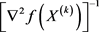${d}^{\left(k\right)}=-{\left[{\nabla }^{2}f\left({X}^{\left(k\right)}\right)\right]}^{-1}\nabla f\left({X}^{\left( k \right)}\right)$

${p}^{\left(k\right)}={H}_{k+1}\cdot {q}^{\left( k \right)}$

(1) ${d}^{\left(k\right)}=-{H}_{k}\nabla f\left({X}^{\left(k\right)}\right)$ 是目标函数 $f\left(X\right)$ 在点 ${X}^{\left(k\right)}$ 的下降方向；

(2) ${H}_{k+1}$ 满足 ${p}^{\left(k\right)}={H}_{k+1}\cdot {q}^{\left(k\right)}$

(3) ${H}_{k+1}$ 与之间应具有某种简单的迭代关系

${H}_{k+1}={H}_{k}+{E}_{k}$

(4) 若有 $\nabla f{\left({X}^{\left(k\right)}\right)}^{\text{T}}\left[-{H}_{k}\nabla f\left({X}^{\left(k\right)}\right)\right]>0$，即 $\nabla f{\left({X}^{\left(k\right)}\right)}^{\text{T}}{H}_{k}\nabla f\left({X}^{\left(k\right)}\right)<0$ 成立，则拟牛顿方向 ${d}^{\left(k\right)}=-{H}_{k}\nabla f\left({X}^{\left(k\right)}\right)$ 必是下降方向。

${E}_{k}={\alpha }_{k}{u}^{\left(k\right)}{u}^{\left(k\right)\text{T}}+{\beta }_{k}{v}^{\left(k\right)}{v}^{\left(k\right)\text{T}}$

$\begin{array}{l}{\alpha }_{k}=\frac{1}{{u}^{\left(k\right)\text{T}}{q}^{\left(k\right)}}=\frac{1}{{p}^{\left(k\right)\text{T}}{q}^{\left(k\right)}}\\ {\beta }_{k}=\frac{-1}{{v}^{\left(k\right)\text{T}}{q}^{\left(k\right)}}=\frac{-1}{{q}^{\left(k\right)\text{T}}{H}_{k}{q}^{\left( k \right)}}\end{array}$

${E}_{k}=\frac{{p}^{\left(k\right)}{p}^{\left(k\right)\text{T}}}{{p}^{\left(k\right)\text{T}}{q}^{\left(k\right)}}-\frac{{H}_{k}{q}^{\left(k\right)}{q}^{\left(k\right)\text{T}}{H}_{k}}{{q}^{\left(k\right)\text{T}}{H}_{k}{q}^{\left( k \right)}}$

$\mathrm{min}f\left(X\right)=-2{x}_{1}{x}_{2}-2{x}_{2}+{x}_{1}^{2}+2{x}_{2}^{2}$

$\nabla f\left(X\right)=\left(\begin{array}{c}2{x}_{1}-2{x}_{2}\\ -2{x}_{1}-2+4{x}_{2}\end{array}\right)$

${d}^{\left(1\right)}=-{H}_{1}{g}_{1}=-\left(\begin{array}{cc}1& 0\\ 0& 1\end{array}\right)\left(\begin{array}{c}0\\ -2\end{array}\right)=\left( 0 2 \right)$

${X}^{\left(1\right)}={\left(0,0\right)}^{\text{T}}$ 出发，沿方向 ${d}^{\left(1\right)}$ 进行一维搜索：

$\underset{t\ge 0}{\mathrm{min}}f\left({X}^{\left(1\right)}+t{d}^{\left( 1 \right)}\right)$

${X}^{\left(2\right)}={X}^{\left(1\right)}+{t}_{1}{d}^{\left(1\right)}=\left(\begin{array}{c}0\\ 0\end{array}\right)+\frac{1}{4}\left(\begin{array}{c}0\\ 2\end{array}\right)=\left(\begin{array}{c}0\\ \frac{1}{2}\end{array}\right)$

${p}^{\left(1\right)}={X}^{\left(2\right)}-{X}^{\left(1\right)}={t}_{1}{d}^{\left(1\right)}=\left(\begin{array}{c}0\\ \frac{1}{2}\end{array}\right)$

${q}^{\left(1\right)}={g}_{2}-{g}_{1}=\left(\begin{array}{c}-1\\ 0\end{array}\right)-\left(\begin{array}{c}0\\ -2\end{array}\right)=\left(\begin{array}{c}-1\\ 2\end{array}\right)$

${H}_{2}={H}_{1}+{E}_{1}={H}_{1}+\frac{{p}^{\left(1\right)}{p}^{\left(1\right)\text{T}}}{{p}^{\left(1\right)\text{T}}{q}^{\left(1\right)}}-\frac{{H}_{1}{q}^{\left(1\right)}{q}^{\left(1\right)\text{T}}{H}_{1}}{{q}^{\left(1\right)\text{T}}{H}_{1}{q}^{\left(1\right)}}=\frac{1}{20}\left(\begin{array}{cc}16& 8\\ 8& 9\end{array}\right)$

${d}^{\left(2\right)}=-{H}_{2}{g}_{2}=-\frac{1}{20}\left(\begin{array}{cc}16& 8\\ 8& 9\end{array}\right)\left(\begin{array}{c}-1\\ 0\end{array}\right)=\frac{1}{5}\left( 4 2 \right)$

${X}^{\left(2\right)}={\left(0,\frac{1}{2}\right)}^{\text{T}}$ 出发，沿方向 ${d}^{\left(2\right)}$ 进行一维搜索：

$\underset{t\ge 0}{\mathrm{min}}f\left({X}^{\left(2\right)}+t{d}^{\left( 2 \right)}\right)$

${X}^{\left(3\right)}={X}^{\left(2\right)}+{t}_{2}{d}^{\left(2\right)}=\left( 1 1 \right)$

DFP方法由于不需要在迭代点处计算Hessin矩阵的逆，所以在极值点附近的收敛速度比牛顿法更快，效率更高。可由表4列出的数值实验可以看出，当初始点距离极值点很远时，受迭代误差的影响，DFP方法很难收敛到极值点。因此，为了提升收敛速度和求解的准确性我们同样可以在迭代点距离极值点很近时再使用拟牛顿方法求解问题。本文将此种改进方法称作GNN算法。Table 4. Initial value points and iteration table of the test function were solved by the DFP methodsTable 5. Initial value points and iteration table of the test function were solved by the GNN methods

5. 物流配送中心选址问题Table 6. Information table of the demand points

$\underset{\left(x,y\right)\in D}{\mathrm{max}}\text{}F\left(x,y\right)=\omega \underset{i=1}{\overset{n}{\sum }}{p}_{i}\left(x,y\right)\cdot {v}_{i}=\omega \underset{i=1}{\overset{n}{\sum }}\frac{1}{1+\underset{j=1}{\overset{m}{\sum }}{\text{e}}^{-\lambda \left({d}_{ij}-{d}_{i}\right)}}\cdot {v}_{i}$，其中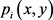表示新建配送中心 $\left(x,y\right)$ 分担需

$\begin{array}{l}{d}_{ij}=\sqrt{{\left({a}_{i}-{x}_{j}\right)}^{2}+{\left({b}_{i}-{y}_{j}\right)}^{2}};\\ {d}_{i}=\sqrt{{\left(x-{a}_{i}\right)}^{2}+{\left(y-{b}_{i}\right)}^{2}}\end{array}$

$\omega =1,\lambda =0.5$，于是该问题可表示为如下非线性规划 ：

$\underset{\left(x,y\right)\in D}{\mathrm{max}}\text{}F\left(x,y\right)=\underset{i=1}{\overset{8}{\sum }}\frac{1}{1+\underset{j=1}{\overset{2}{\sum }}{\text{e}}^{-\frac{1}{2}\left({d}_{ij}-{d}_{{}_{i}}\right)}}{v}_{i}$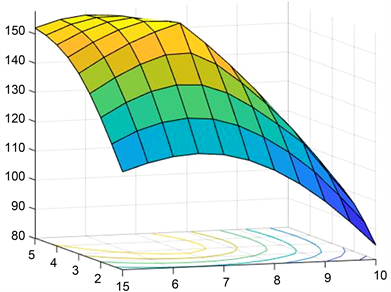Figure 5. Function image of the objective function for the logistics distribution center problemTable 7. Comparative analysis table of the five methods

6. 结论

NOTES

*通讯作者。

 陈宝林. 最优化理论与算法[M]. 北京: 清华大学出版社, 2005.

 甘应爱. 运筹学: 本科版[M]. 北京: 清华大学出版社, 2005.

 马昌凤. 最优化方法及其Matlab程序设计[M]. 北京: 科学出版社, 2010.

 Huff, D.I. (1964) Defining and Estimating a Trading Area. Journal of Marking, 28, 34-38.
https://doi.org/10.1177/002224296402800307

 Drezner, T. (1994) Locating a Single New Facility among Existing Unequally Facilities. Journal of Regional Science, 34, 237-252.
https://doi.org/10.1111/j.1467-9787.1994.tb00865.x

 王瑞, 胡洁琼. 基于竞争的配送中心选址研究[J]. 物流科技, 2013(7): 54-55+60.

Top